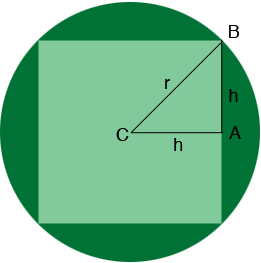SEARCH HOMEMath Central Quandaries & QueriesQuestion from Jenn: Hello! I am about to buy a 7'9" round rug, but I want to have it cut down into a square. What's the largest square I can obtain from this? Thank you!Hi Jenn,

I am not sure which dimension of the circle is $7'9" = 7.75'$ but I'll show you how to find the side length $s$ of the circle if you know $r,$ the radius.If $h$ is half the the side length if the square as in the diagram then, since triangle ABC is a right triangle Pythagoras' theorem tell us that

$h^2 + h^2 = r^2 \mbox{ or } 2 h^2 = r^2 .$

Thus $s = 2 h = \sqrt{2} r.$

I hope this helps,
PennyMath Central is supported by the University of Regina and The Pacific Institute for the Mathematical Sciences.